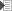# 3.1 Notation

First we need to determine how we're going to express algebraic equations and scientific units and their symbols and prefixes.

## Equations

In the equations these standard mathematical operators are used:

 x·y multiplication x/y division xy x raised to the power of y 10log x the 10-base logarithm of x

## Units and symbols

In electricity, just as in any other physics subdepartment, quantities have a name, symbol, unit and unit symbol. An example that speaks for itself: time. The quantity's name is time, the symbol is t, the unit is second and the unit symbol is s.

 Quantity Symbol Unit Unit symbol Voltage V Volt V Current I Ampère A Power P Watt W Resistance R Ohm Ω Frequency f Hertz Hz

## Prefixes

When values become very large or very small, we can decide to put a prefix in front of the unit symbol, to indicate the magnitude of the number. That way we don't have to write down all the zeros that precede or trail the value. The most common prefix is kilo, as in kilogram (kg), kiloWatt (kW), kiloHertz (kHz). Below is a list of the most common prefixes and their multipliers. A more elaborate list can also be found in the appendix. Note that these prefixes are case-sensitive.

 Prefix Symbol Power Absolute micro μ 10-6 .000001 milli m 10-3 .001 centi c 10-2 .01 deci d 10-1 .1 - - 100 1 kilo k 103 1,000 mega M 106 1,000,000

© Joris van den Heuvel 2001-2009